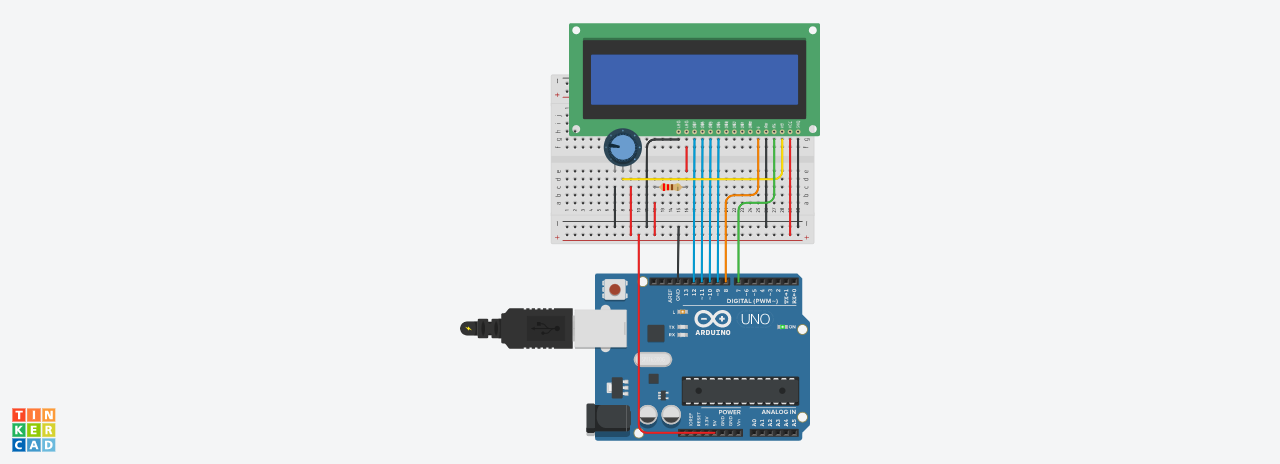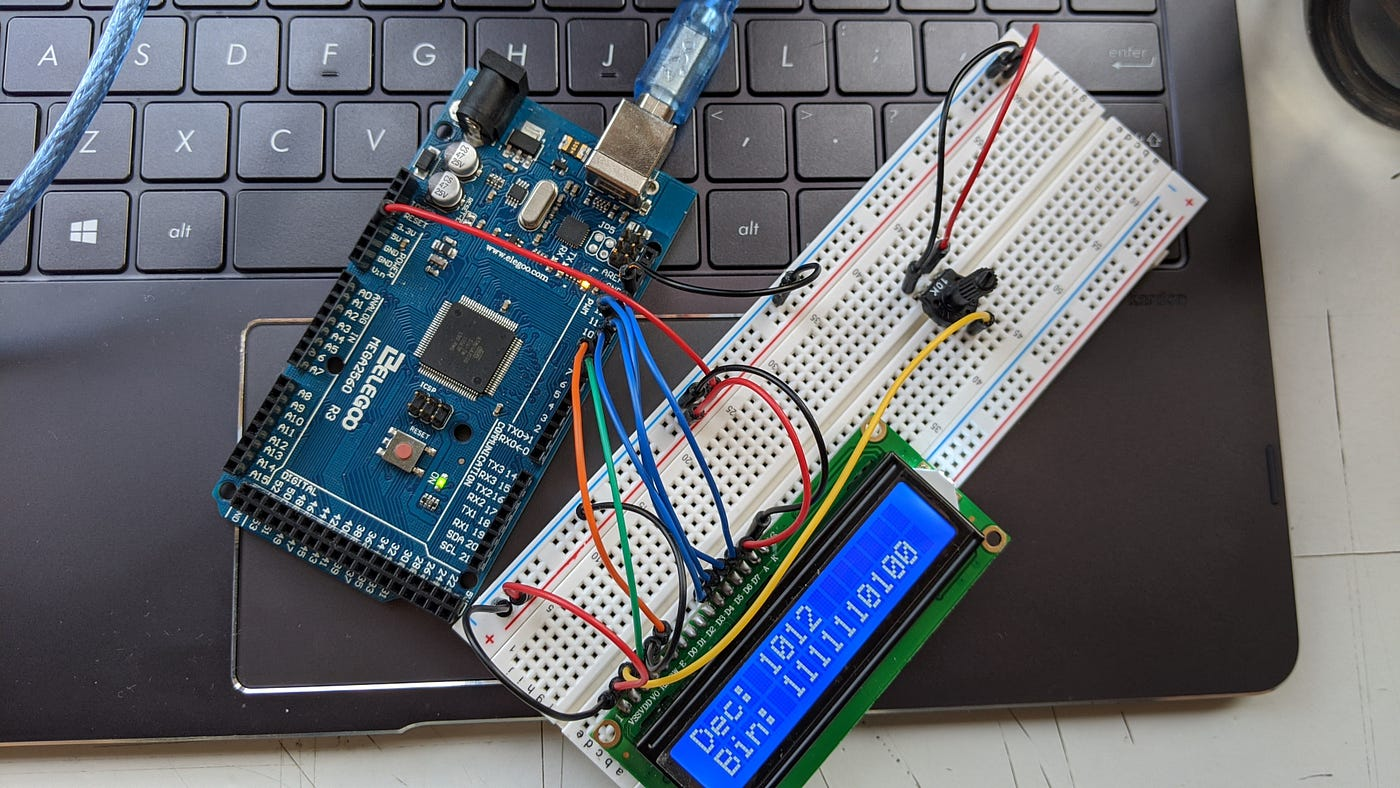# Create a decimal-to-binary calculator with Arduino

As an Engineering student, I have to deal with problems related to binary numbers. Digital circuits involve many calculations with binary numbers: logic gates, flip flops, and particularly Analog to Digital Converters. Thus, I created an Arduino program that helps to understand how to convert a decimal number (integer) into a binary number. Additionally, I used an LCD screen display along with the Arduino microcontroller to display the input and output numbers.

# The hardware

For this project, I used an Arduino Mega 2560, based on the ATmega2560 microcontroller. Connected to the Arduino, there’s an LCD screen to display the input and output values, as illustrated in [Fig. 1].Fig. 1: Circuit design with the Arduino UNO and the LCD screen.

Due to software limitations, I used the Arduino UNO instead of the MEGA 2560 for the design, but the I/O pins are the same for both.

## The LCD screen

The LCD screen pins are shown in [Fig. 2].

The GND pin goes to the negative power rail (ground). VCC stands for “voltage at the common collector,” and goes to the positive power rail (5-V supply from the Arduino). The V0-pin is for screen-contrast and is connected to a 1-kOhm potentiometer’s output. The LCD requires a potentiometer to adjust the contrast of the LCD.

RS stands for Register Select and goes connected to pin 7 of the Arduino. If the Arduino outputs a 0, then the LCD will read it as a command; if it outputs a 1, however, it reads the data to display on the LCD. The RW pin designates the Read/Write input; since we are writing data to the LCD, the pin goes to the negative power rail (digital 0). The E (enable) pin goes to pin 8; it latches the data on the data pins D0 to D7, to show whatever data we want on the screen.

The LCD screen can be used either with 4 (D4 to D7) or 8 bits (D0 to D7); since we are using the latter, the LCD screen will operate with 4 bits. Lastly, LED1 and LED2 go to the positive and negative power rails, respectively, which are used for turning on a blacklight LED built in the LCD. As you can see in [Fig. 1], there is a 220-Ohm resistor connected across LED1 and the 5-V rail. This is because the software showed an error message declaring that too much current was going into the LCD screen. However, the current provided by the Arduino MEGA from the 5-V output is 400-mA and is not enough to damage the LCD.

# The software

## Set-up the LCD screen

To program the LCD, we need to include the LiquidCrystal.h library. A library is a collection of resources that provide extra functionality for use in sketches . To program the LCD, we need to include the LiquidCrystal.h library. A library is a collection of resources that provide extra functionality for use in sketches . On the other hand, we need to declare the variable type “LiquidCrystal” to tell the Arduino to which pins we are connecting the LCD. In this case, we are using pins 7 (RS), pin 8 (E), and pins 9 to 12 (D4 to D7).

## void setup

The void loop is called and runs only one after we power up the Arduino or reset it.  It is used to initialize variables, pin modes, libraries, etc. For this program, we will initialize the lcd variable to use 16 columns (vertically) and 2 rows (horizontally). Serial.begin(9600) initializes the Serial Monitor, where we are to output some variables, and the 9600 means we are exchanging data with the Serial Monitor at a data rate of 9600 bits per second . Finally, the program calls the displayMenu(); subroutine, which we’ll discuss later.

## void loop

The void loop loops continually after void setup has been called .

First, we initialize a boolean variable, testNumber, to identify if the input number is less than 1023. I chose 1023 because it’s the higher number the LCD can display in binary using the program’s configuration. Then, the program calls the subroutine getNumber(); and whatever number the user types is saved in decimal_Number.

Then, the program will call the verifyNumber(); subroutine and will test if the number is less than 1023. Depending on the result, it will store true or false in testNumber. The last step is the if statement; if the number is less than 1023, i.e. testNumber is true, it will convert it to binary. After that, the program repeats itself.

The function illustrated in [Fig. 6] displays, “Insert a number up to 1023.” The void statement means the function will return no data to the previous function that calls it . In other words, displayMenu() won’t return anything to the loop() subroutine discussed in the preceding section.

lcd.begin(16,2), as discussed before, fixes the display for 16 columns and 2 rows. Similarly, lcd.setCursor(0,0) positions the cursor to the top left corner of the LCD, and setCursor(0,1) to the bottom left corner . We will use the lcd.print(); statement throughout the project to print the results on the LCD. We will see later that we can print both text and integer values using this method.

## getNumber

Unlike displayMenu();, getNumber() returns an integer value to be stored in decimal_Number (see [Fig. 5]). The while statement is important: Serial.available == 0 means that the program will wait until the user inputs a number. If the user writes a number, though, it will be stored in decimalNumber using Serial.parseInt();. It is essential to know that this statement reads only integers values from the Serial Monitor.

## verifyNumber

As the number implies, this subroutine verifies that the number is less than or equal to 1023. Note the function returns a boolean value, thus, true or false, 1 or 0. If decimal_Number is less than or equal to 1023, the program clears the LCD and prints “Dec: ” plus whatever decimal number the user wrote. Then, it stores true in testNumber (see [Fig. 5]).

However, if the number is greater than 1023, the LCD shows the following text “Enter a number <= 1023” and stores false in testNumber.
Note that for this function, we both send values (inputNumber a.k.a. decimal_Number) and return a different value type (bool testNumber).

## convertToBinary

For this subroutine, we will need an array of integer numbers. An array is a group of variables, which have an index number . The size of this array is 10 (the index numbers will go from 0 to 9). This is because the higher binary number we will calculate has 10 bits.

To convert a decimal number to a binary number, we need two variables: the reminder and the quotient. The remainder is the number we are going to store in the binary_Number array. The quotient, on the other hand, is the number the program will keep dividing until it fills the 10 positions of binary_Number. That is what the for loop does: i = 0, and each time it repeats itself, it will add 1 to i until it gets to 9 (i < 10). After performing all the calculations, the program sets the cursor at the bottom left corner, and prints “Bin: ”.

Note that the remainder of 1 and 2, i.e., 1%2, equals 1.

When the program saved the remainders in binary_Number, i.e., the new binary number, those were saved backward. For example, 10 equals to 1010 in binary, but the program saves the remainders as follows: 0101. Thus, we need to reorder the array. The for loop does that work: it starts at 9, the last position of the array. Therefore, each time the for loop repeats, it will print the last number, position 9, as the first bit, position 0, and so on. In other words, if we had 10110 initially, after the for loop, we will see 01101 on the LCD.

On the other hand, lcd.setCursor(cursorPlace + 5, 1); takes care of the 5 spaces “Bin: ” occupies, and prints it in the bottom left corner. Also, each time the program prints a number of the array, cursorPlace moves the cursor one place to the right: cursorPlace++.

# The final resultFig. 10: actual circuit using the Arduino MEGA and the LCD screen.

As you can see, that’s how my circuit looks like. In this case, it is displaying decimal 1012, and the equivalent in binary 1111110100. Of course, I am not a Computer Science major, and coding is just a hobby to me. There is always room for improvement, and I encourage you to boost and improve my code. If you have any commentary or doubts, I will be more than pleased to read and answer all your comments.

References:

1. “Arduino-Libraries.” Arduino. https://www.arduino.cc/en/reference/libraries (accessed April 7, 2020).
2. “setup().” Arduino. https://www.arduino.cc/reference/en/language/structure/sketch/setup/ (accessed April 7, 2020).
3. “begin().” Arduino. https://www.arduino.cc/en/Serial.Begin (accessed April 7, 2020).
4. “loop().” Arduino. https://www.arduino.cc/reference/en/language/structure/sketch/loop/ (accessed April 7, 2020).
5. “void.” Arduino. https://www.arduino.cc/reference/tr/language/variables/data-types/void/ (accessed April 7, 2020).
6. “setCursor() method.” Arduino. https://www.arduino.cc/en/Tutorial/LiquidCrystalSetCursor (accessed April 7, 2020).
7. “array.” Arduino. https://www.arduino.cc/reference/tr/language/variables/data-types/array/ (accessed April 7, 2020).

--

--